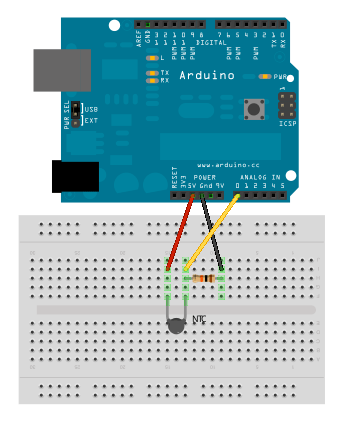## Environment – NTC ThermistorNTC thermistor is a type of resistor whose resistance varies with temperature. It can be used in the same way as any other variable resistor such as LDRs, flex sensors etc. It is extremely easy to use and cheap, at least if you don’t need ISO-standard-quality accuracy.

The lab thermistors are 10 K NTC resistors. With an accuracy of ??? And with which kind of Steinhart-Hart coefficient values? (A, B, and C in the formula below are the Steinhart-Hart coefficients which vary depending on the type and model of thermistor) Will have to check this, don’t remember now where we bought them. — edit: I got the values but they don’t work? lets see, Antti got this working in the Bodyfit project, I’ll try to post the values here soon. Anyhow, it seems to give consistant values (related to SHT15 temperatures) when using the arduino code below, copied from Arduino Playground. (there is also a longer code with more explanations and possibilities for displaying the data:

```// Utilizes the Steinhart-Hart Thermistor Equation:
//    Temperature in Kelvin = 1 / {A + B[ln(R)] + C[ln(R)]^3}
//    where A = 0.001129148, B = 0.000234125 and C = 8.76741E-08
#include <math.h>

double Temp;
// Assuming a 10k Thermistor.
//Calculation is actually: Resistance = (1024 * BalanceResistor/ADC) - BalanceResistor
Temp = 1 / (0.001129148 + (0.000234125 * Temp) + (0.0000000876741 * Temp * Temp * Temp));
Temp = Temp - 273.15;            // Convert Kelvin to Celcius
//in case you need farenheit:
// Temp = (Temp * 9.0)/ 5.0 + 32.0; // Convert Celcius to Fahrenheit
return Temp;
}

void setup() {
Serial.begin(115200);
}

void loop() {